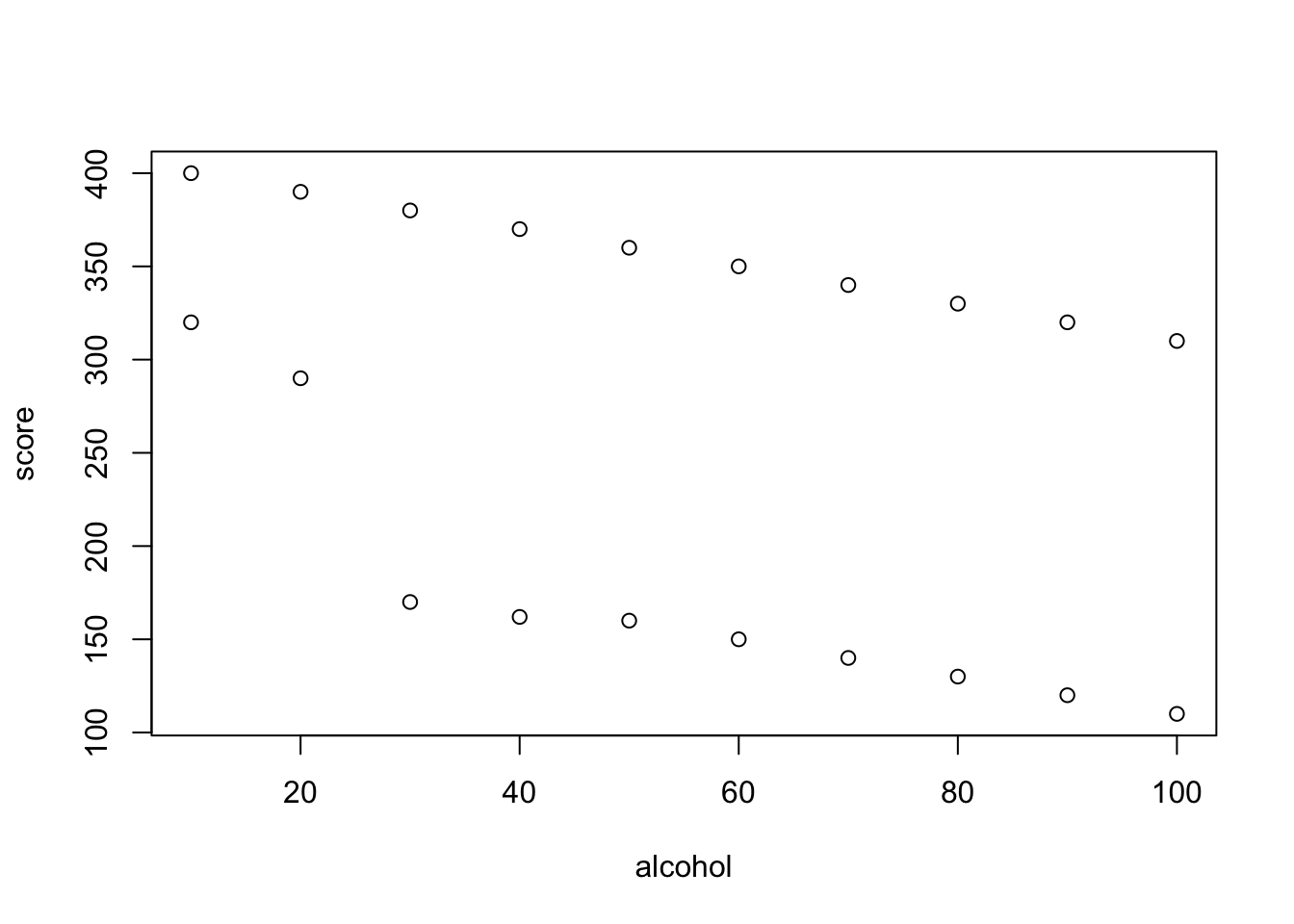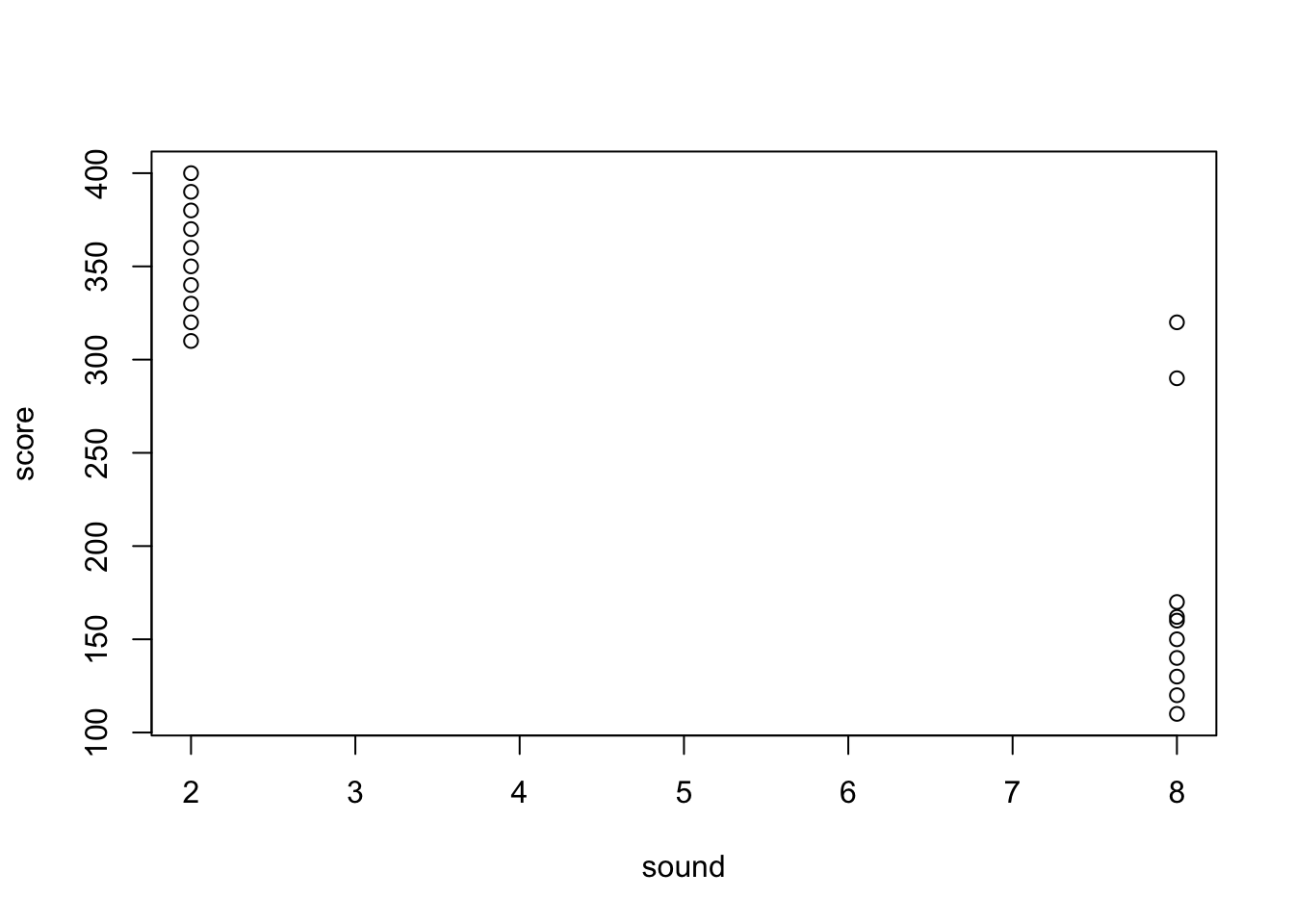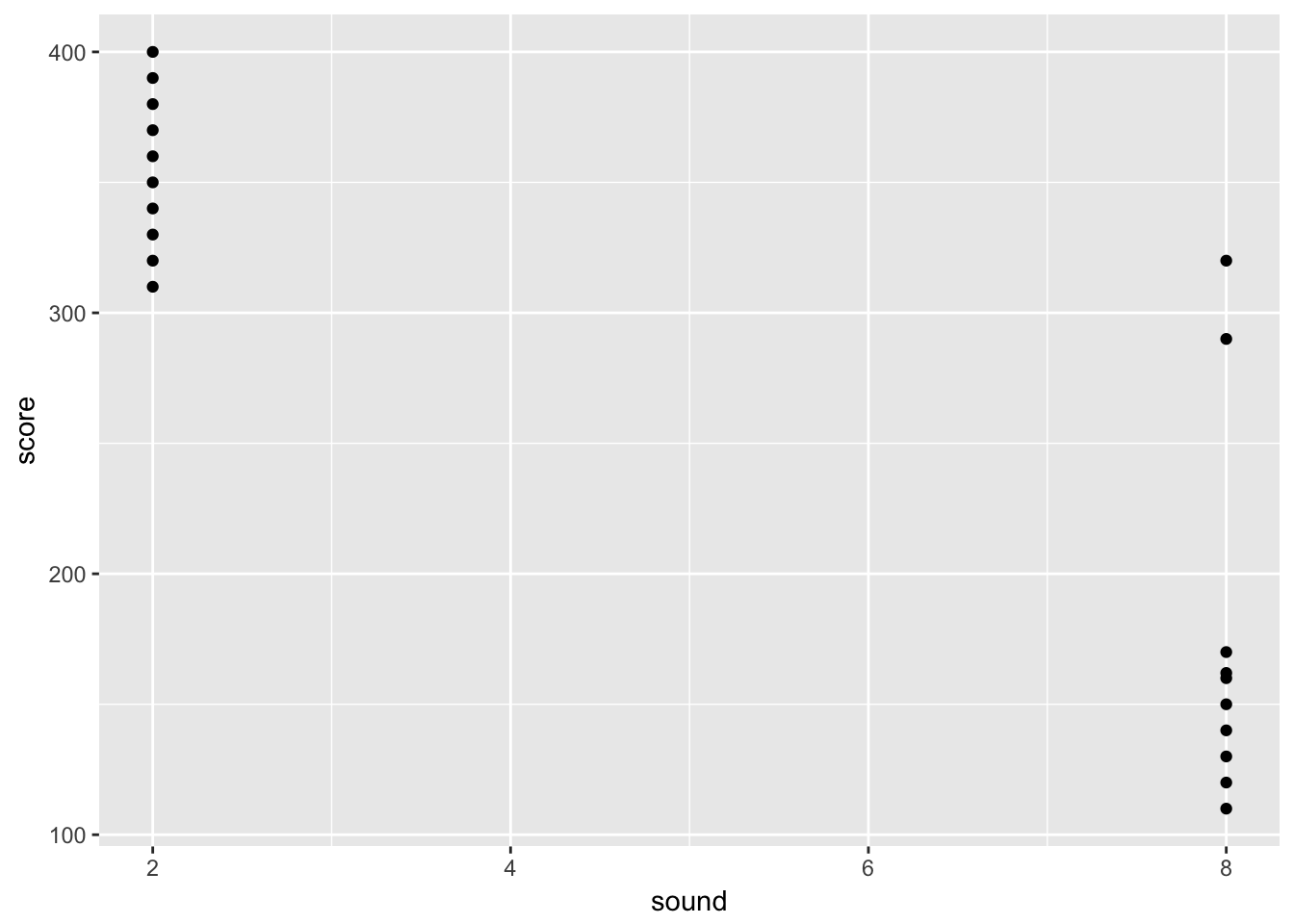Examining the relationship between one predictor and one outcome is fun. But there’s much more fun to be had! Most models examine the relationship between an outcome and multiple predictors.

Why look at multiple predictors? Well, let us consider our hypothetical alcohol example. We measure game score after people drank different amounts of alcohol. What is the music at the bar was at different volumes for different people?

## Another example

This time we’ll have 30 people. Each person is given a drink with some alcohol in it. They go into a soundproof room where we play music at one of 2 different volumes. We will have one person in each alcohol and sound condition.

This will make more sense with the data.

``````rm(list=ls())
df.as <- data.frame(
alcohol = c(10, 20, 30, 40, 50, 60, 70, 80, 90, 100, 10, 20, 30, 40, 50, 60, 70, 80, 90, 100),
sound = c(2,2,2,2,2,2,2,2,2,2,8,8,8,8,8,8,8,8,8,8),
score = c(400, 390, 380, 370, 360, 350, 340, 330, 320, 310, 320, 290, 170, 162, 160, 150, 140, 130, 120, 110))``````

## Seeing is believing

To see what is happening lets visualise the data.

We could use the graphics built into R (as we have before). These base graphics take the regression formula as an argument!

``plot(score ~ alcohol + sound, data = df.as)``What do you think? Does the volume of the sound influence the score? What about alcohol? In fact, or is there a combined effect? So many questions.

Perhaps we should start by exploring the data more. The ggplot package is a nice way to visualise data. It is quite different from base graphics so there is no pressure to learn this.

We can plot the data as follows in ggplot2.

``````#install.packages('ggplot2')
require('ggplot2')``````
``## Loading required package: ggplot2``
``````ggplot(data = df.as, aes(x = sound, y = score)) +
geom_point()````````````ggplot(data = df.as, aes(x = sound, y = score, group = sound)) +
geom_boxplot()``````